# The Most Common Digital SAT Math Topics: Part 2

0
55In the first installment of this series, we covered four of the eight most common digital SAT math topics: Finding an Equation to Fit the Data, Exponential Functions, Finding Intercepts, and Quadratics. This time we’ll look at the remaining four: Solving Equations, Systems of Equations, Linear Relationships, and Evaluating Functions at a Given Value

Solving Equations

Most of us are familiar with the process of solving an equation: find the value of the variable that makes the equation true. Most students can solve the most basic version of this problem in 10 seconds.

We typically use inverse operations to isolate our variable. In this case, we’d subtract 30 from both sides to cancel out the + 30 next to the x:That was easy. But sometimes we’ll see trickier versions of this concept, like this.The basic strategy is still the same: isolate the variable, which happens to be w this time, solving for its value. One thing to note: we’re not asked to solve for w, but rather for 2w. So we’ll need to be careful about that. Let’s distribute the 3 into the parentheses, combine like terms, and find w, which we can then use to find the value of 2w: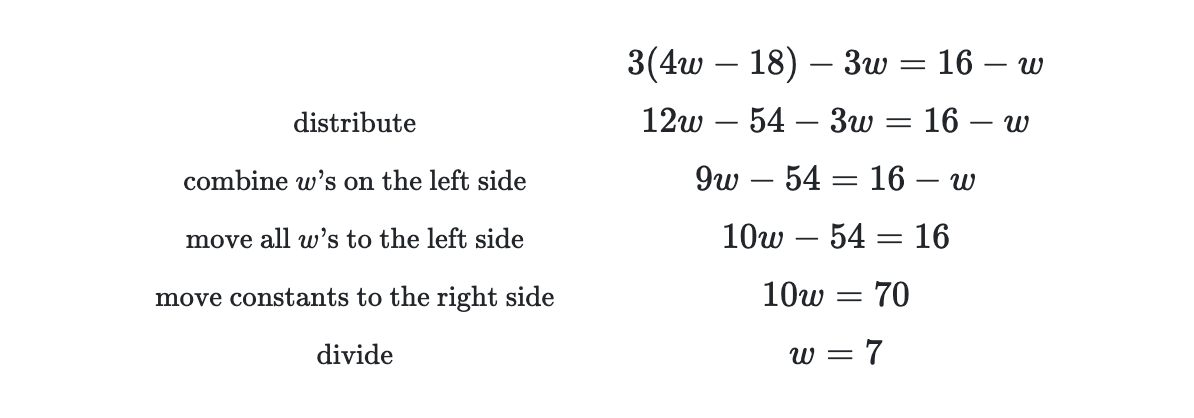Since w is 7, 2w will be 2(7), which is 14, our final answer. One last thing: we can always test the value we get for the variable by plugging it back into the original equation to make sure the equation works. So we can plug in 7 to replace w in the original equation, and both sides of the equation will equal the same number, showing us that our answer is correct.

Systems of Equations

As we touched on in our post on the power of Desmos, a system of equations is just two equations that we’re looking at simultaneously; commonly, we’ll need to find that system’s solution, another name for the intersection points of their graphs. Here’s an example: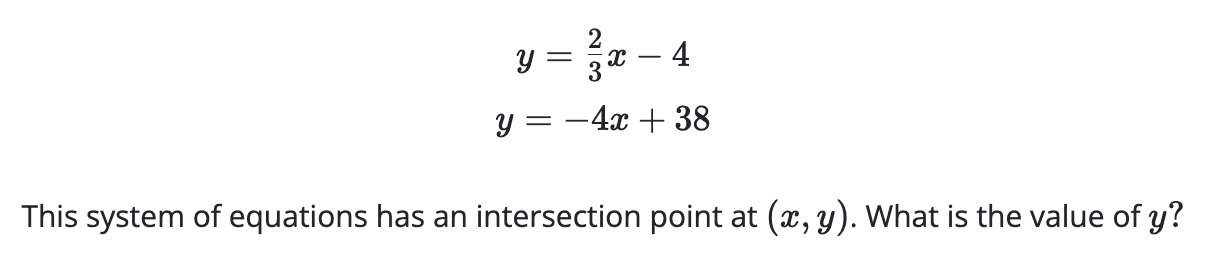Notice that they want not the (x, y) coordinates of the intersection point, but just the y-value there. The quickest way to do most systems of equations questions is to use Desmos, so let’s use it here: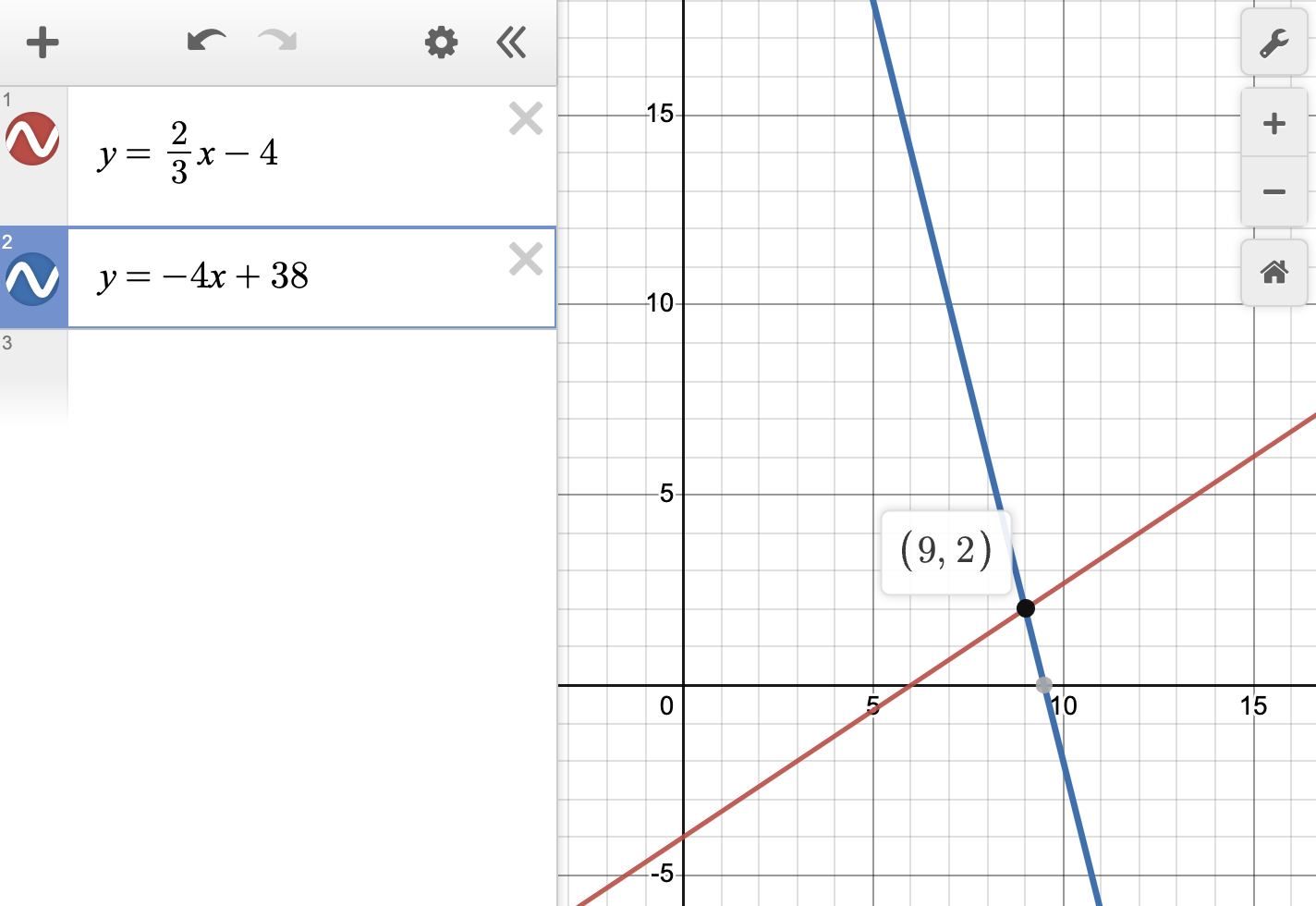So the y-value at the intersection point is 2, our answer. You can certainly use algebra to solve this one, but doing so will take significantly longer for most students than simply using Desmos.

Linear Relationships

Any given situation can be modeled by a linear relationship if it consists of two variables, one of which changes at a constant rate for each unit change in the other variable. For example, if a loaf of bread costs \$4, there’s a linear relationship between the total cost and how many loaves of bread are purchased: each additional loaf adds \$4 to the total cost. However, there’s not a linear relationship between the population of the world and the number of years since 2000, for example, since population growth is exponential, not linear: the population doesn’t grow by the same number of people each year.

How can we see this concept on the SAT? Here’s a common type of question: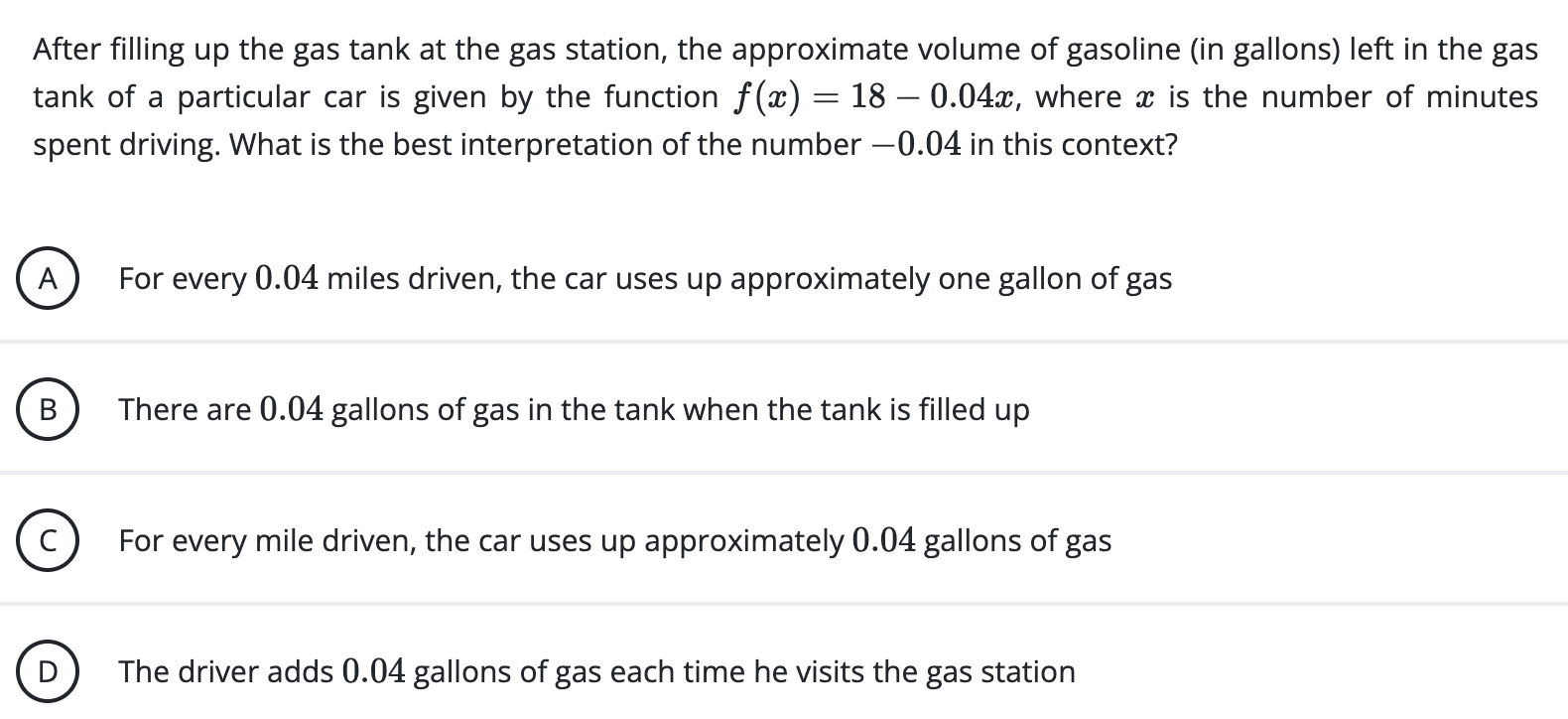The given equation might remind us of the equation y = mx + b, where m is the slope of the line and b is its y-intercept. The only difference is that the order of mx and b is reversed, but that won’t matter. 18 would be the y-intercept of this line, the starting number of gallons of gas when x = 0 and no minutes have been driven since filling the gas tank. So the gas tank must have 18 gallons of gas in it when it’s full. Now, let’s consider the –0.04 part: notice that this is being multiplied by x, our time in minutes, so the total gallons of gas must drop by 0.04 gallons every minute; this is why this number is being multiplied by x. And 0.04 is negative because the amount of gas goes down as the car is driven. This matches up with the explanation given in C, which is our answer.

Evaluating Functions at a Given Value

Functions are usually given to us using function notation: f(x) = 2x + 1, for instance. That f(x), pronounced “f of x,” essentially means here’s the function with x plugged in. So here, 2x + 1 would be the function. If we’re asked to evaluate the function at a given value, we’ll just take out the x in the original function and replace it with whatever number they give us. So f(-2), for example, means replacing x in the function with -2, while f(0) means plugging in 0 for x.

On the SAT, we could certainly see something like f(-2), which would make evaluating the given function simple. However, sometimes we aren’t given that same notation and will still need to know that we have to plug a value into the function: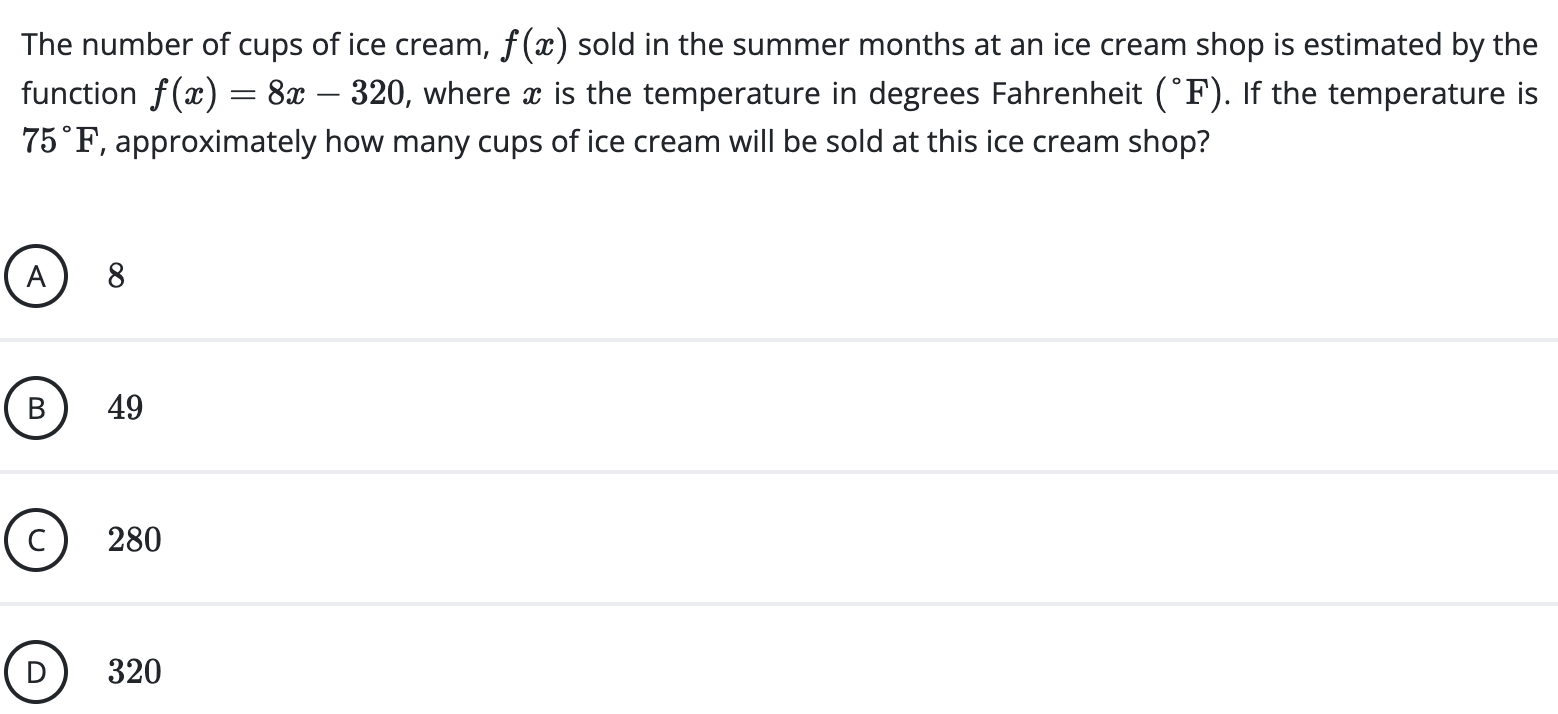Notice that they don’t specifically use the notation of f(1) or f(2) or anything similar, which would make this one slightly more manageable. But they do give us a certain temperature, 75oF, and ask us how many cups of ice cream will be sold at this temperature. What’s the key here? 75 oF is the temperature, x, and the number of cups of ice cream sold is f(x). So they’re really asking us to find f(75); in other words, just plug 75, our x-value, in place of x in the function:So we get C: 280 cups of ice cream.

As with anything, practice is the key to getting your goal score on the SAT, and it involves taking official practice tests, familiarizing yourself with the question types, and focusing on getting answers quickly. As we’ve shown, the eight most-tested topics are ones that are relatively easy to master and that can sometimes be solved using Desmos. On test day, if you can get all of the questions right for these eight most common topics, you’ll have already gotten about half of the entire math section correct!

If you find yourself needing more material than just the College Board’s practice tests, or if you’d like detailed lessons on each SAT topic, check out our self-paced SAT course in Methodize, with guided lessons and hundreds of practice questions and explanations for finding each answer.